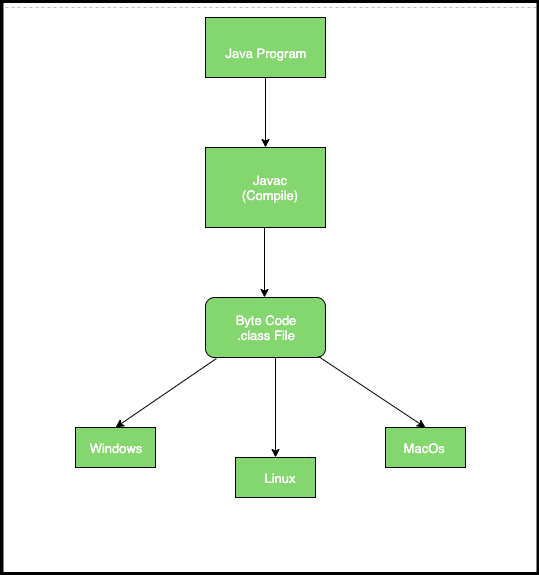Open in App
Not now

# Why is Java ‘write once and run anywhere’?

• Difficulty Level : Basic
• Last Updated : 26 May, 2019

JVM(Java Virtual Machine) acts as a run-time engine to run Java applications. JVM is the one that actually calls the main method present in Java code. JVM is a part of the JRE(Java Runtime Environment).

Java applications are called WORA (Write Once Run Anywhere). This means a programmer can develop Java code on one system and can expect it to run on any other Java-enabled system without any adjustment. This is all possible because of JVM.

How Java is WORA:

In traditional programming languages like C, C++ when programs were compiled, they used to be converted into the code understood by the particular underlying hardware, so If we try to run the same code at another machine with different hardware, which understands different code will cause an error, so you have to re-compile the code to be understood by the new hardware.

In Java, the program is not converted to code directly understood by Hardware, rather it is converted to bytecode(.class file), which is interpreted by JVM, so once compiled it generates bytecode file, which can be run anywhere (any machine) which has JVM( Java Virtual Machine) and hence it gets the nature of Write Once and Run Anywhere.Example: Practical Implementation of WORA using a simple JAVA program to check whether a number is even or odd.

 `import` `java.util.Scanner;`` ` `class` `GFG {``    ``public` `static` `void` `main(String args[])``    ``{``        ``int` `num;``        ``System.out.println(``"Enter a number:"``);``        ``Scanner input = ``new` `Scanner(System.in);``        ``num = input.nextInt();``        ``if` `(num % ``2` `== ``0``)``            ``System.out.println(num + ``" is even"``);``        ``else``            ``System.out.println(num + ``" is odd"``);``    ``}``}`

Conclusion:
To sum it up, Java, when compiled, creates a bytecode (.class file), which can be run in any machine which supports JVM. So once compiled it doesn’t require re-compilation at every machine it runs, JVM converts the bytecode to be understood by the underlying hardware.

My Personal Notes arrow_drop_up